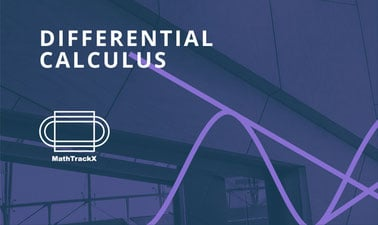# MathTrackX: Differential Calculus

Discover concepts and techniques relating to differentiation and how they can be applied to solve real world problems.## There is one session available:

After a course session ends, it will be archived.
Estimated 4 weeks
3–6 hours per week
Self-paced

This course is part three of the MathTrackX XSeries Program which has been designed to provide you with a solid foundation in mathematical fundamentals and how they can be applied in the real world.

This course will cover basic concepts and techniques relating to differentiation; a fundamental tool of calculus. Derivatives are key to the understanding of rates of change, that is the extent to which a function responds to changes in a dependent variable.

Guided by experts from the School of Mathematics and the Maths Learning Centre at the University of Adelaide, this course will cover concepts and techniques to provide a foundation for the applications of differentiation in STEM related careers and/or further study at the undergraduate level.

Join us as we provide opportunities to develop your skills and confidence in applying mathematics to solve real world problems.

### At a glance

• Subject: Math
• Level: Introductory
• Prerequisites:
None
• Language: English
• Associated programs:

# What you'll learn

Skip What you'll learn
• How derivatives relate to the slope of a function

• How to calculate the derivative of polynomials and special functions

• Differentiation rules for evaluating the derivative of non-trivial functions

• The concept and application of ‘the second derivative’

• How to use differentiation to solve problems in basic motion, mechanics and optimisation.